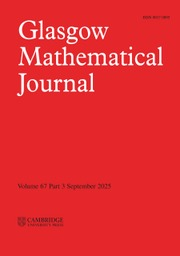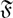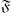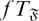Home
Hostname: page-component-544b6db54f-lmg95 Total loading time: 0.169 Render date: 2021-10-19T09:32:00.380Z Has data issue: true Feature Flags: { "shouldUseShareProductTool": true, "shouldUseHypothesis": true, "isUnsiloEnabled": true, "metricsAbstractViews": false, "figures": true, "newCiteModal": false, "newCitedByModal": true, "newEcommerce": true, "newUsageEvents": true }Glasgow Mathematical Journal

# ON GENERALISED PRONORMAL SUBGROUPS OF FINITE GROUPS

Published online by Cambridge University Press:  22 August 2014

## Abstract

HTML view is not available for this content. However, as you have access to this content, a full PDF is available via the ‘Save PDF’ action button.

For a formation$\mathfrak F$, a subgroup M of a finite group G is said to be$\mathfrak F$-pronormal in G if for each g ∈ G, there exists x ∈ 〈U,Ug$\mathfrak F$ such that Ux = Ug. Let f be a subgroup embedding functor such that f(G) contains the set of normal subgroups of G and is contained in the set of Sylow-permutable subgroups of G for every finite group G. Given such an f, let fT denote the class of finite groups in which f(G) is the set of subnormal subgroups of G; this is the class of all finite groups G in which to be in f(G) is a transitive relation in G. A subgroup M of a finite group G is said to be$\mathfrak F$-normal in G if G/CoreG(M) belongs to$\mathfrak F$. A subgroup U of a finite group G is called K-$\mathfrak F$-subnormal in G if either U = G or there exist subgroups U = U0U1 ≤ . . . ≤ Un = G such that Ui–1 is either normal or$\mathfrak F$-normal in Ui, for i = 1,2, …, n. We call a finite group G an$fT_{\mathfrak F}$-group if every K-$\mathfrak F$-subnormal subgroup of G is in f(G). In this paper, we analyse for certain formations$\mathfrak F$ the structure of$fT_{\mathfrak F}$-groups. We pay special attention to the$\mathfrak F$-pronormal subgroups in this analysis.

## Keywords

Type
Research Article
Information
Glasgow Mathematical Journal , September 2014 , pp. 691 - 703

## References

1.Ballester-Bolinches, A., Beidleman, J. C., Feldman, A. D. and Ragland, M. F., On generalised subnormal subgroups of finite groups, Math. Nachr. 286 (11–12) (2013), 10661071.CrossRefGoogle Scholar
2.Ballester-Bolinches, A., Esteban-Romero, R. and Asaad, M., Products of finite groups, De Gruyter Expositions in Mathematics, vol. 53 (Springer, Berlin, Germany, 2010) 584 pp.CrossRefGoogle Scholar
3.Ballester-Bolinches, A. and Ezquerro, L. M., Classes of finite groups, Mathematics and Its Applications (Springer, Berlin, Germany, 2006), 584 pp.Google Scholar
4.Ballester-Bolinches, A., Feldman, A. D., Pedraza-Aguilera, M. C. and Ragland, M. F., A class of generalised finite T-groups, J. Algebra 333 (1) (2011), 128138.CrossRefGoogle Scholar
5.Ballester-Bolinches, A. and Pérez-Ramos, M. D., On$\mathfrak F$-subnormal subgroups and Frattini-like subgroups of a finite group, Glasgow Math. J. 36 (1994), 241247.CrossRefGoogle Scholar
6.Beidleman, J. C. and Heineken, H., Finite soluble groups whose subnormal subgroups permute with certain classes of subgroups, J. Group Theory 6 (2003), 139158.CrossRefGoogle Scholar
7.Bryce, R. A. and Cossey, J., The Wielandt subgroup of a finite soluble group, J. London Math. Soc. 40 (1989), 244256.CrossRefGoogle Scholar
8.Doerk, K. and Hawkes, T., Finite soluble groups (De Gruyter, Berlin, Germany, 1992).CrossRefGoogle Scholar
9.Feldman, A.,$\mathfrak F$-Bases and subgroup embedding in finite solvable groups, Arch. Math. 47 (1986), 481492.CrossRefGoogle Scholar
10.Feldman, A. D., t-groups and their generalizations, Group theory (Granville, OH, 1992) (World Sci. Publ., River Edge, NJ, 1993), 128133.Google Scholar
11.Müller, N.,$\mathfrak F$-pronormale untergruppen endlich auflösbarer gruppen. (Johannes Gutenberg-Universität Mainz, Diplomarbeit, 1985).Google Scholar
12.Peng, T. A., Finite groups with pro-normal subgroups, Proc. Amer. Math. Soc. 20 (1969), 232234.CrossRefGoogle Scholar
13.Robinson, D. J. S.A note on finite groups in which normality is transitive, Proc. Amer. Math. Soc. 19 (1968), 933937.CrossRefGoogle ScholarYou have Access
1
Cited by

# Send article to Kindle

Note you can select to send to either the @free.kindle.com or @kindle.com variations. ‘@free.kindle.com’ emails are free but can only be sent to your device when it is connected to wi-fi. ‘@kindle.com’ emails can be delivered even when you are not connected to wi-fi, but note that service fees apply.

Find out more about the Kindle Personal Document Service.

ON GENERALISED PRONORMAL SUBGROUPS OF FINITE GROUPS
Available formats
×

# Send article to Dropbox

To send this article to your Dropbox account, please select one or more formats and confirm that you agree to abide by our usage policies. If this is the first time you use this feature, you will be asked to authorise Cambridge Core to connect with your <service> account. Find out more about sending content to Dropbox.

ON GENERALISED PRONORMAL SUBGROUPS OF FINITE GROUPS
Available formats
×

# Send article to Google Drive

To send this article to your Google Drive account, please select one or more formats and confirm that you agree to abide by our usage policies. If this is the first time you use this feature, you will be asked to authorise Cambridge Core to connect with your <service> account. Find out more about sending content to Google Drive.

ON GENERALISED PRONORMAL SUBGROUPS OF FINITE GROUPS
Available formats
×
×